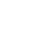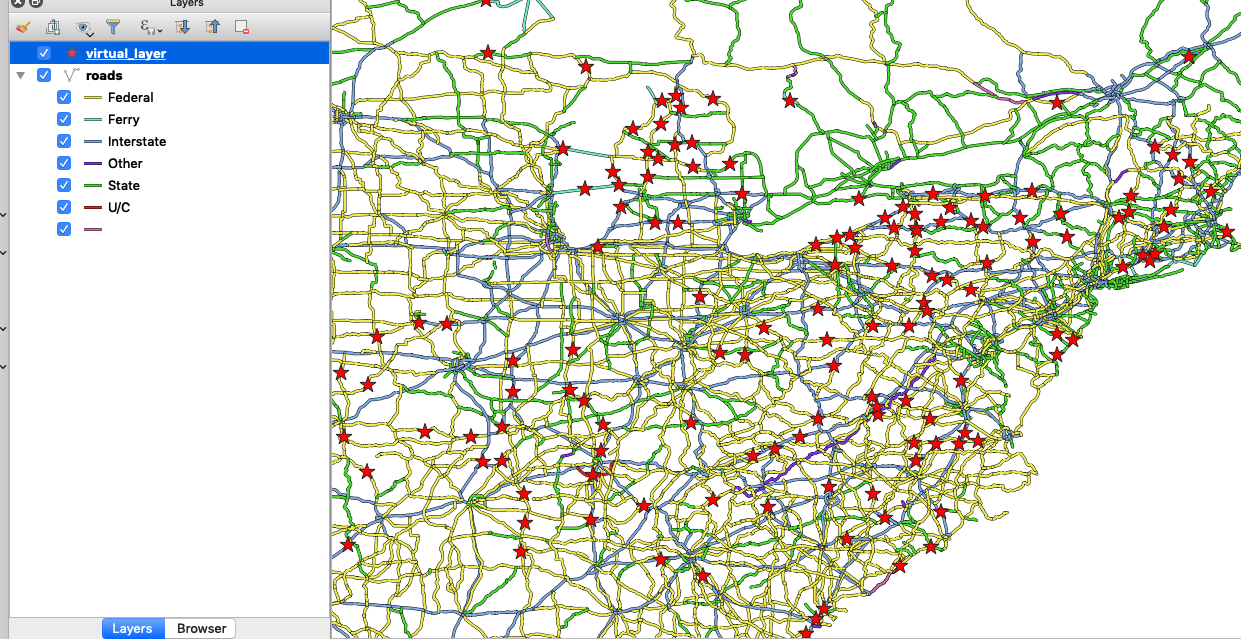### Advanced Attribute expressions in Context

“The attribute table does not expose the geometry column but expressions allow you to manipulate it and generate new geometry.”

Attribute defines the characteristics of an entity. The attribute table view is limited to showing tabular data and excludes showing the geometry column. The geometry is only exposed in expression and users can manipulate it to generate the area, length and do geoprocessing.
In this module, we explore how to construct expressions to manipulate the geometry of layers regardless of their visibility in the attribute table.#### You try:

Goal: To manipulate the geometry of layers using geometry expressions.

• Add the layers from the exercise data.
• Toggle edit the roads layer.
• Use the field calculator to create two new columns called latitude and longitude. Ensure the data types for the columns are decimal and precision 3.
• Update the latitude column with Midpoint x expression.
• Update the longitude column with Midpoint y expression.
• Save your edits and toggle edit off.
NB We need to generate a point layer using the two columns we have populated above. There are two solutions to this.

Solution A

• Activate the processing toolbox
• Use the search menu in the processing toolbox to search for the algorithm Create Points Layer from Table
• In the algorithm select the X, Y to match the longitude, latitude columns
• Symbolize the point layer as you see fit.

Solution B

• Create a virtual layer.
• Import the roads layer
• Use the Point expression.### More about geometry expressions

Attribute data describes the characteristics of features. Expressions provide a means to manipulate these attribute to derive new information or to update existing data. Geometry expressions allow layer geometry to be manipulated to generate new geometry or to update the existing geometry. The most common expression allow deriving new information like area, length and perimeter. In QGIS you can use the expression to generate new attributes that are of the different geometry type. From a polygon, you can derive the centroids and store them in the attribute table. Just like in the exercise we manipulated the geometry to extract the midpoint of the line geometry.